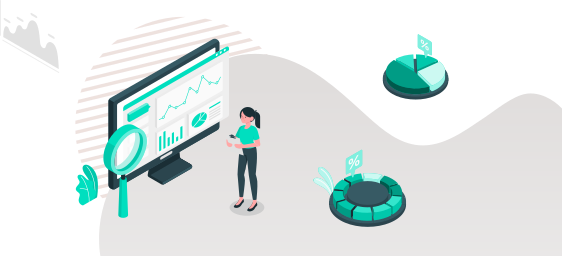Últimos

New features:

## Machine Learning

New methods:

• Model.plot: draws a decomposition (PCA & SVD) scatter plot
• Model.plot_circle: draws a decomposition circle

## Preprocessing

New methods:

• vDataFrame.balance: balances the vDataFrame using the input response column
• vDataFrame.cdt: computes the complete disjunctive table

## Statistical Tests

• New function: cochrane_orcutt performs a Cochrane-Orcutt estimation, adjusting linear models for serial correlation